## ScintPack SA

#### Description

The ScintPack Laboratory Data Acquisition, Evaluation and Reporting System provides a means to acquire, visualize, evaluate and otherwise reduce to useful form, data captured from a wide variety of laboratory instruments.

Its modular design partitions the users approach to organized data collection and maintenance, into four general categories.

#### Data Acquisition

ASCII data records, sometimes called data frames, are captured from the data source. The individual data elements within each record are parsed using a data frame template mask. Only the data elements matching the mask are placed in the data viewer as data points on a trace.

A data source may be one of the following:
• Laboratory instruments
• Data loggers
• Computers
• ASCII data files on disk
• SP-100
• Keyboard entry

Data are collected and processed in one of three ways.

### Time Series

The data is represented as a variable with respect to time. This form of data acquisition is most common wherever chemical or physical processes are measured or monitored over a finite time period.

Four Data Types can be processed and applied to a Time Series and are characterized as follows.

#### Differential Data

Differential processing uses the difference between two measurements or two points in time as a measure of the quantity of interest. This form of processing is useful when monitoring PressVol / Temp relationships.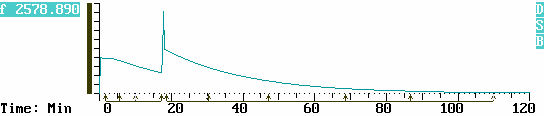In depth TRI-SORBER application note

#### Simple Data

Non-cumulative data where the data values directly represent the quantity of interest. Examples of Simple data would be Temperature or pH measurements.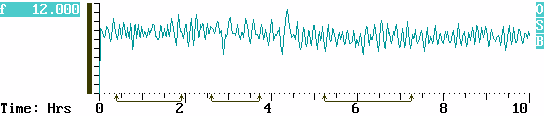#### Integral Data

A quantitative determination can be derived by calculating the Area under the curve.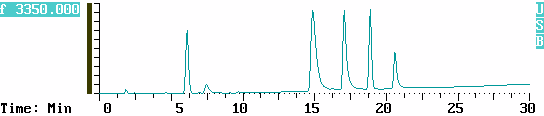Radiochemical data is a form of Integral but with the results expressed in CPM or DPM, the common units of radioactivity.### Counter Mode Data

The data represents a pre-processed output from another data system which has already made measurements and calculated results. Counter Mode data is represented as a sample number such as a vial or test tube. It may be a fractionated sample, either manually pipetted or automatically collected via a fraction collector. In addition this form of data may be entered directly from the Keyboard.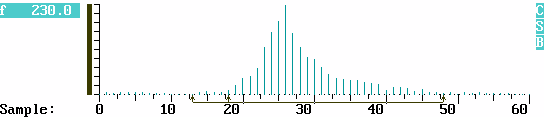### Histogram

Statistical data is stored and displayed as a frequency distribution where measured values are plotted against frequency of occurrence. Histogram Mode is used wherever a measurement is made over and over in order to gain a statistical measure of tendency or deviation. Output tables report Range, Mean and Standard Deviations for tagged regions. Typical applications include:

• Quality Control
• Stability and Systematic Error Testing
• Mathematical Modeling
• Group and Population Studies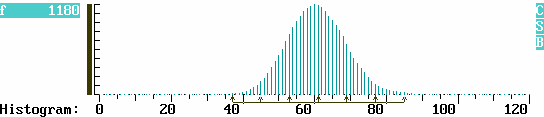Contact Knospler at Scintco